Drop a Query

# Machine Learning## MICE imputation – How to predict missing values using machine learning in Python

MICE Imputation, short for ‘Multiple Imputation by Chained Equation’ is an advanced missing data imputation technique that uses multiple iterations of Machine Learning model training to predict the missing values using known values from other features in the data as predictors. What is MICE Imputation? You can impute missing values by predicting them using other …## Spline Interpolation – How to find the polynomial curve to interpolate missing values

Spline interpolation is a special type of interpolation where a piecewise lower order polynomial called spline is fitted to the datapoints. That is, instead of fitting one higher order polynomial (as in polynomial interpolation), multiple lower order polynomials are fitted on smaller segments. This can be implemented in Python. You can do non-linear spline interpolation …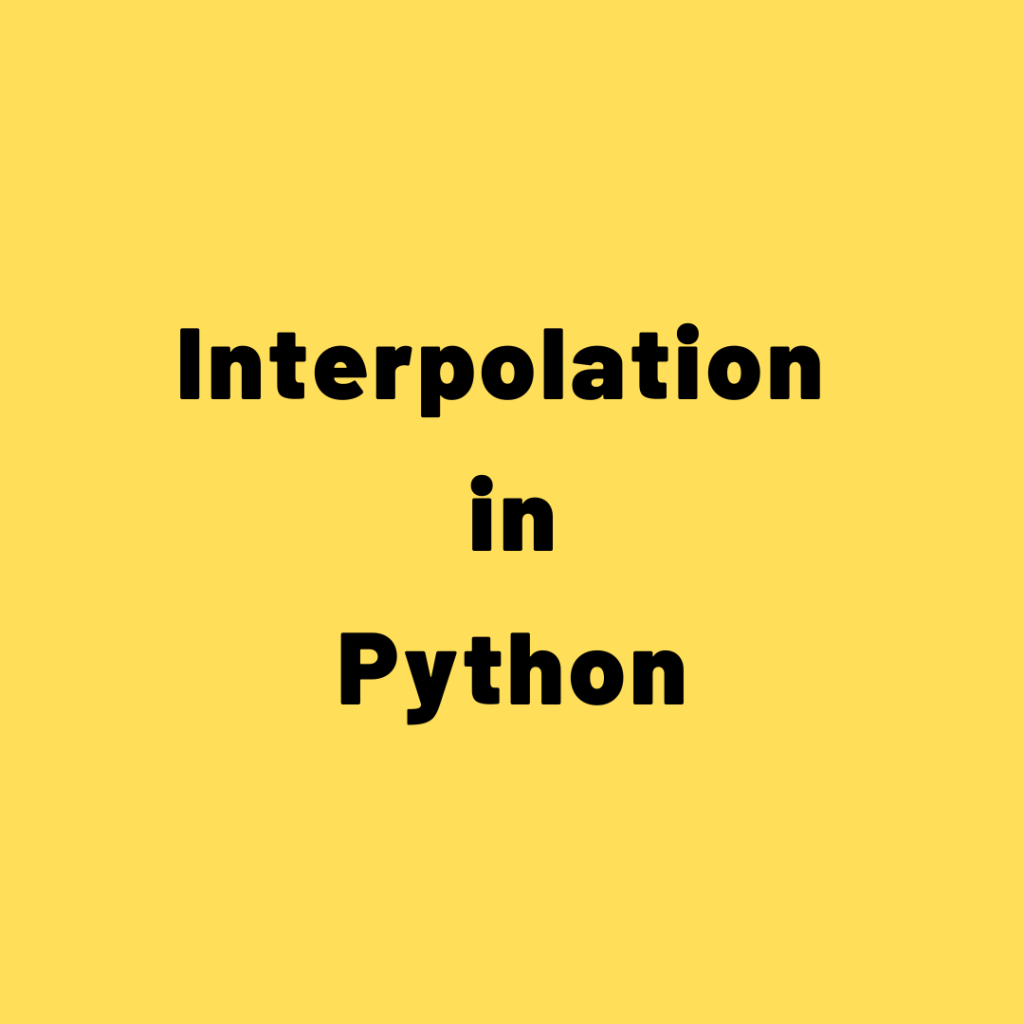## Interpolation in Python – How to interpolate missing data, formula and approaches

Interpolation can be used to impute missing data. Let’s see the formula and how to implement in Python. But, you need to be careful with this technique and try to really understand whether or not this is a valid choice for your data. Often, interpolation is applicable when the data is in a sequence or …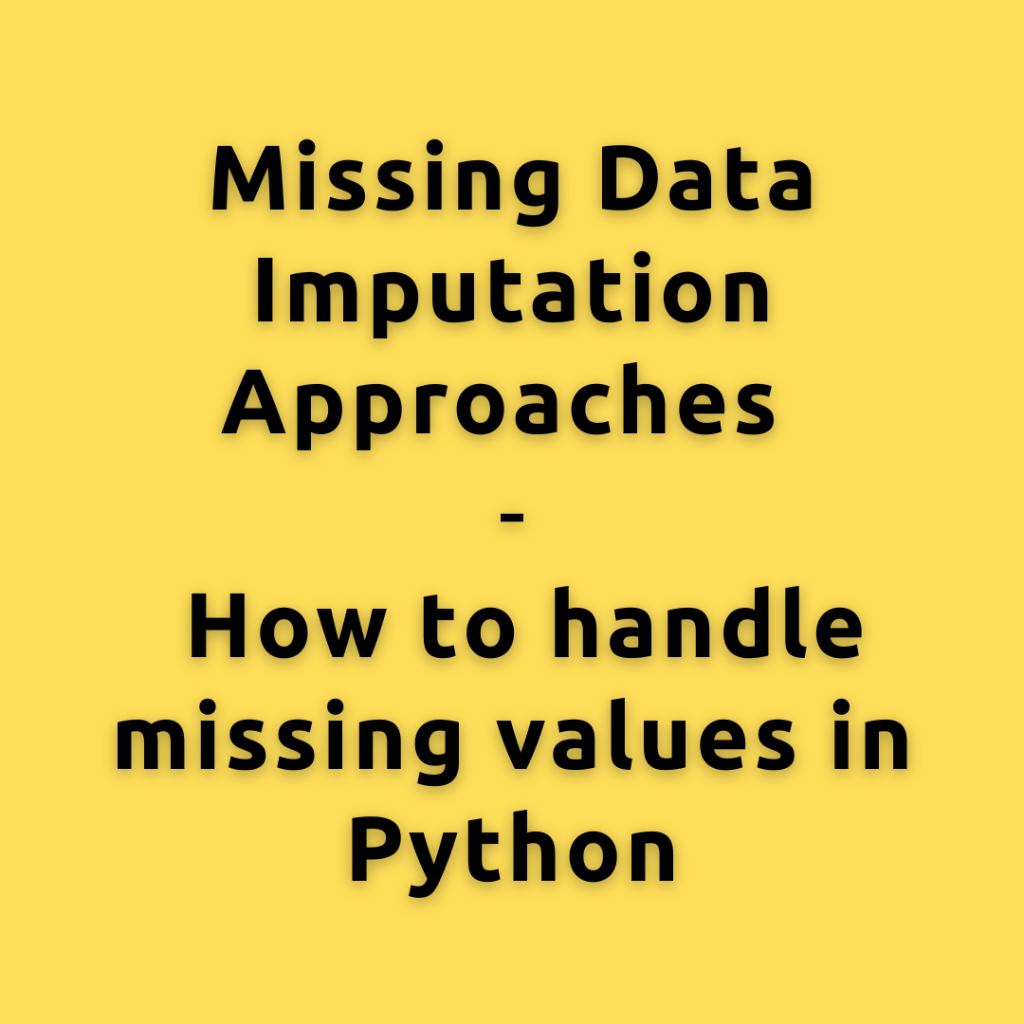## Missing Data Imputation Approaches | How to handle missing values in Python

Machine Learning works on the idea of garbage in – garbage out. If you put in useless junk data to the machine learning algorithm, the results will also be, well, ‘junk’. The quality and consistency of results depend on the data provided. Missing values in data degrade the quality. Why clean the data before training …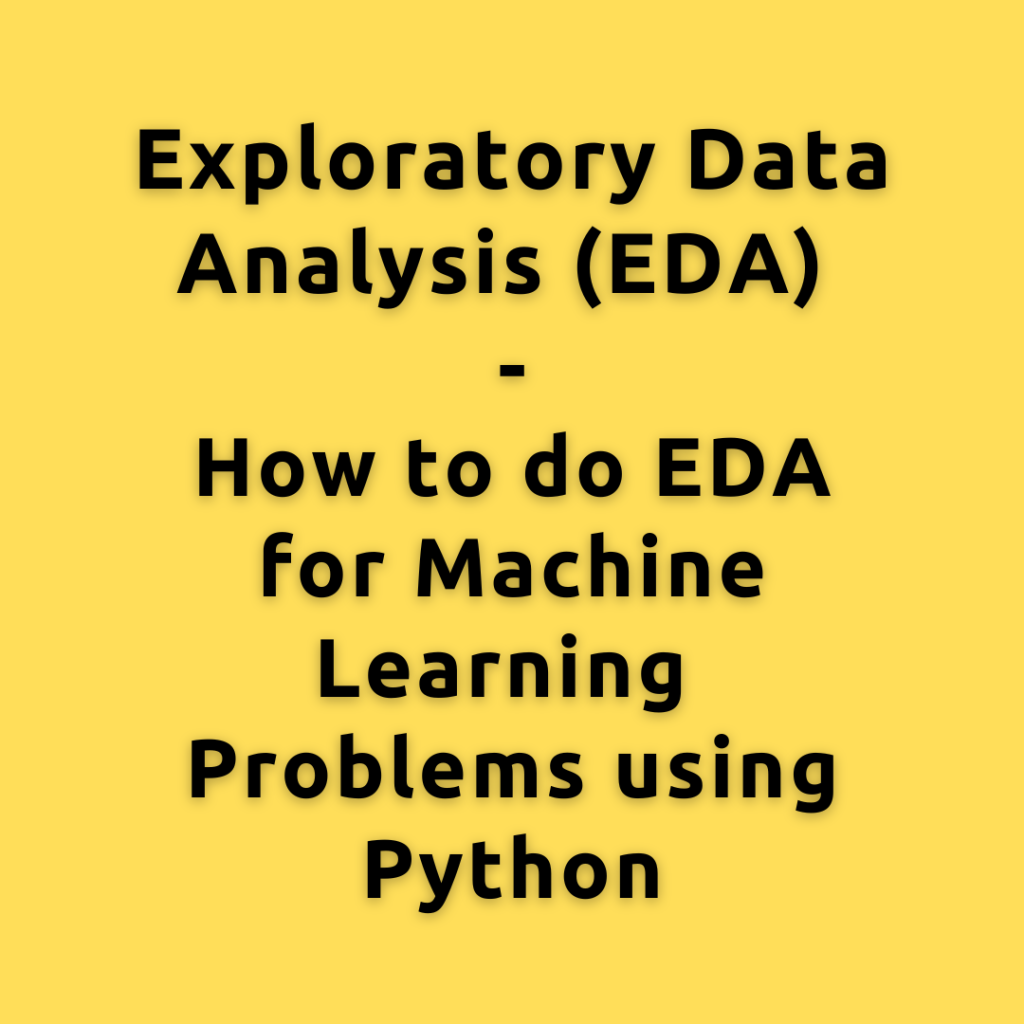## Exploratory Data Analysis (EDA) – How to do EDA for Machine Learning Problems using Python

Exploratory Data Analysis, simply referred to as EDA, is the step where you understand the data in detail. You understand each variable individually by calculating frequency counts, visualizing the distributions, etc. Also the relationships between the various combinations of the predictor and response variables by creating scatterplots, correlations, etc. EDA is typically part of every …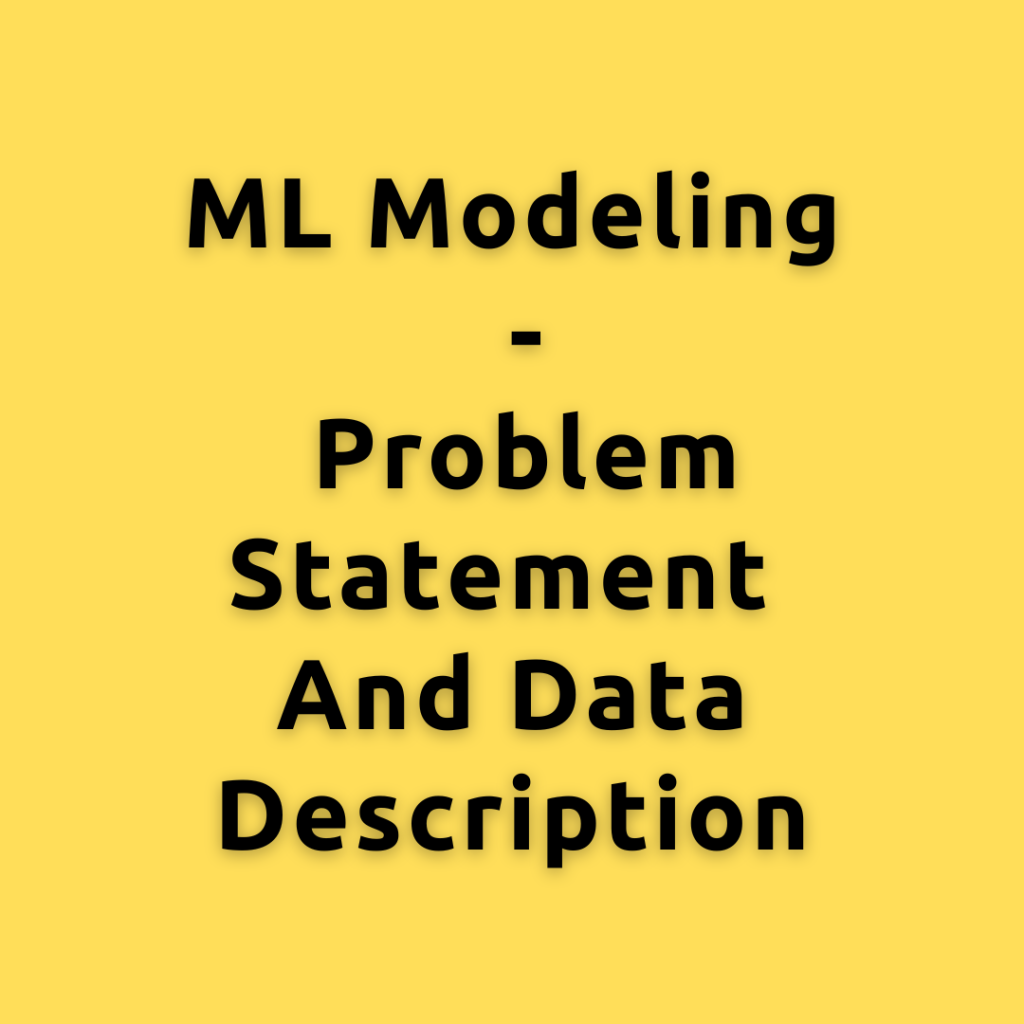## ML Modeling – Problem statement and Data description

ML modeling is the step where machine learning is used to find patterns in data and use that learned knowledge to predict an outcome. The type of ML modeling we are going to solve in this problem is called ‘Churn Modeling’. Let’s first understand the Churn modeling problem statement and then go over the data …Adaboost is one of the earliest implementations of the boosting algorithm. It forms the base of other boosting algorithms, like gradient boosting and XGBoost. This tutorial will take you through the math behind implementing this algorithm and also a practical example of using the scikit-learn Adaboost API. Contents: What is boosting? What is Adaboost? Algorithm …

## How to formulate machine learning problem

Let’s understand how to define and formulate the machine learning problem (for predictive modeling) from a business problem. This structured approach should help you apply the process to most other types of predictive modeling problems at work. Introduction Often in ML teams, you will hear from the business/company departments about the problems and issues they …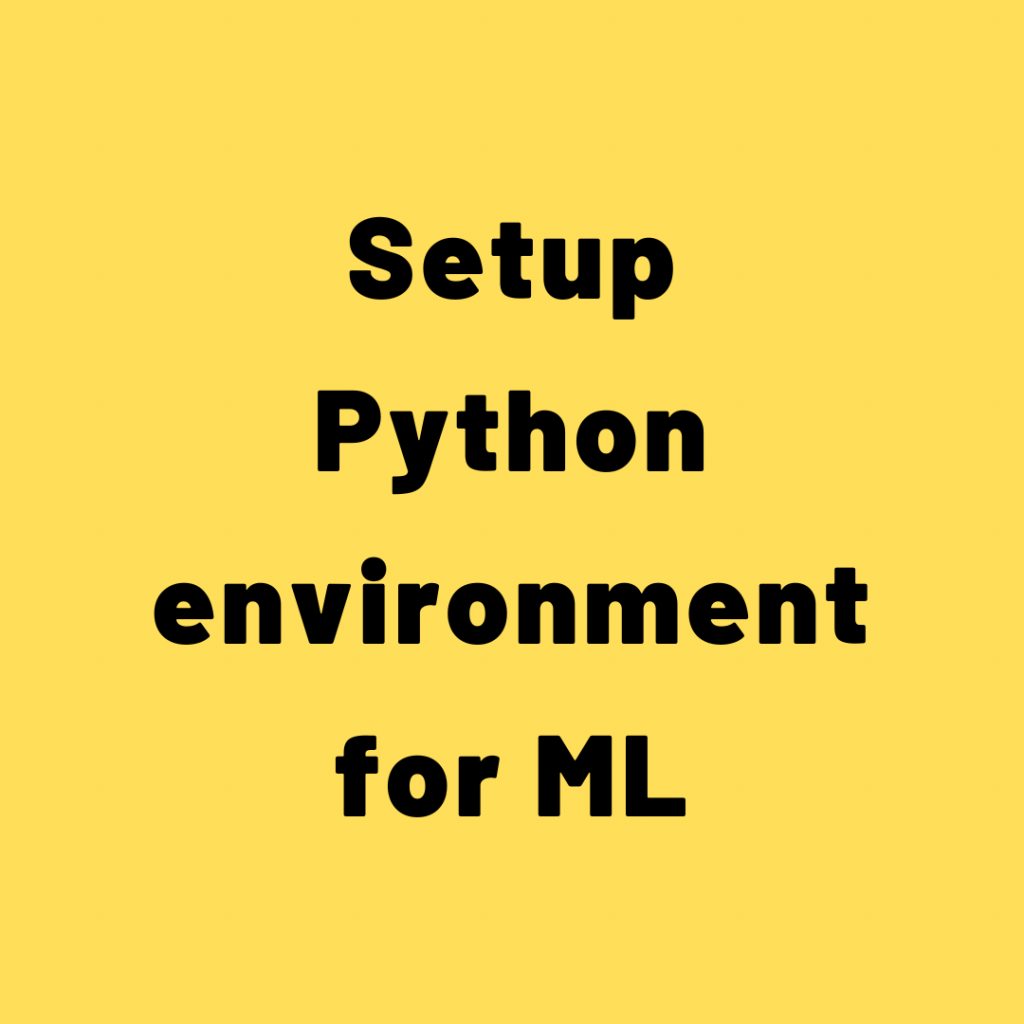## Setup Python environment for ML

Python is the most popular programming language used for AI and machine learning. Let’s see how to setup python environment for ML using anaconda. How to install Python? Simply visit Python.org, go to downloads section, download latest version that shows there and install it like you do for any other software. To do machine learning …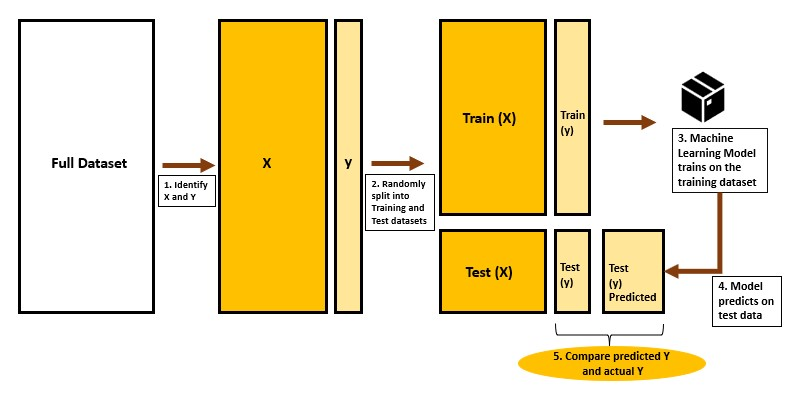## Train Test Split – How to split data into train and test for validating machine learning models?

The train-test split technique is a way of evaluating the performance of machine learning models. Whenever you build machine learning models, you will be training the model on a specific dataset (X and y). Once trained, you want to ensure the trained model is capable of performing well on the unseen test data as well. …

## Task Checklist for Almost Any Machine Learning Project

A cheat sheet of tasks and things to take care of for every end-to-end ML projects. In this, I write down a check list of items and tasks to check whenever you start with a new Data Science / ML project. Once you start off with the project there will be so many things going …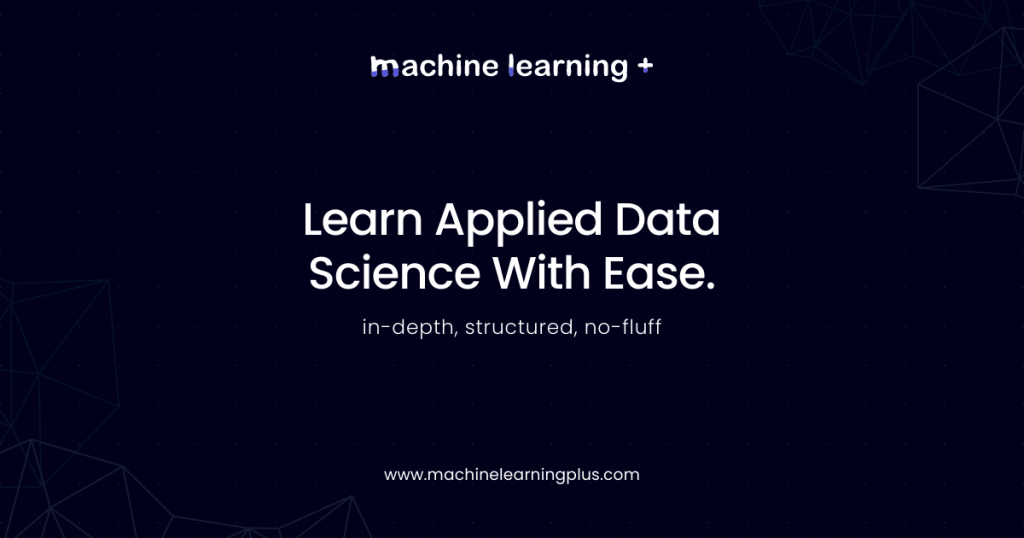## Data Science Roadmap – How to become a Data Scientist? (6 month self study plan)

Today, I discuss the Data Science Roadmap, the missing guide to self study machine learning. I’ll discuss what exactly you need to know and do in order to self study Data science / ML / AI / Stats. I will provide you with some of the best resources for each topic, why you need to …## Why learn the math behind Machine Learning and AI?

Why learn the math behind machine learning algorithms when you can readily implement it using the python libraries like scikit-learn, h2o, statsmodels etc? This is a fair question especially coming from beginners when it is easy to implement ML with few lines of code and get the results fast. Now, you must understand that learning …## Mistakes programmers make when starting machine learning

Today, I want to discuss some of the common mistakes that programmers make when starting to learn machine learning. But first, let me speak about why software engineers should start looking into ML. First, let’s see why programmers should start ML? Today, from what I’ve seen, people coming with a strong software engineering background, good …## Machine Learning Use Cases – The Big List of Real World Applications by Vertical and Industry

The use cases of machine learning to real world problems keeps growing as ML/AI sees increased adoption across industries. However, there are certain core use cases that add lot of value for organizations and you’ll often find them being implemented in banks, healthcare, manufacturing, product companies or by consulting organizations as well. Let’s tour of …## Main Pitfalls in Machine Learning Projects

Not all Data Science projects that get kicked-off see the light of day. While this is true for any project, there are common reasons specific to Data Science projects, which can be avoided. These can either be prevented if you are aware and not commit these mistakes. Or if you see these problems, consider avoiding …

## Regression Coefficient Formula

Let’s understand the formula for the linear regression coefficients. That is the formula for both alpha and the beta. Now, if you have simple linear regression that does, you have just 1x variable in your data, you will be able to compute the values of alpha and beta using this formula. Let’s suppose you …

## Linear Regression in Machine Learning – Clearly Explained

In this lesson, I introduce what Linear regression is all about. Linear Regression is a foundational algorithm for machine learning and statistical modeling. Traditionally, Linear Regression is the very first algorithm you’d learn when getting started with predictive modeling. While there are a lot more ML and Deep learning algorithm in use today, …## Linear Regression in Machine Learning – Clearly Explained

Understanding linear regression. Let’s understand what linear regression is all about from a non-technical perspective, before we get into the details, we will first understand from a layman’s terms what linear regression is. Now, linear regression is a machine learning algorithm ml algorithm that uses data to predict a quantity of interest, typically, we call …Course Preview

## Machine Learning A-Z™: Hands-On Python & R In Data Science

### Free Sample Videos:#### Machine Learning A-Z™: Hands-On Python & R In Data Science#### Machine Learning A-Z™: Hands-On Python & R In Data Science#### Machine Learning A-Z™: Hands-On Python & R In Data Science#### Machine Learning A-Z™: Hands-On Python & R In Data Science